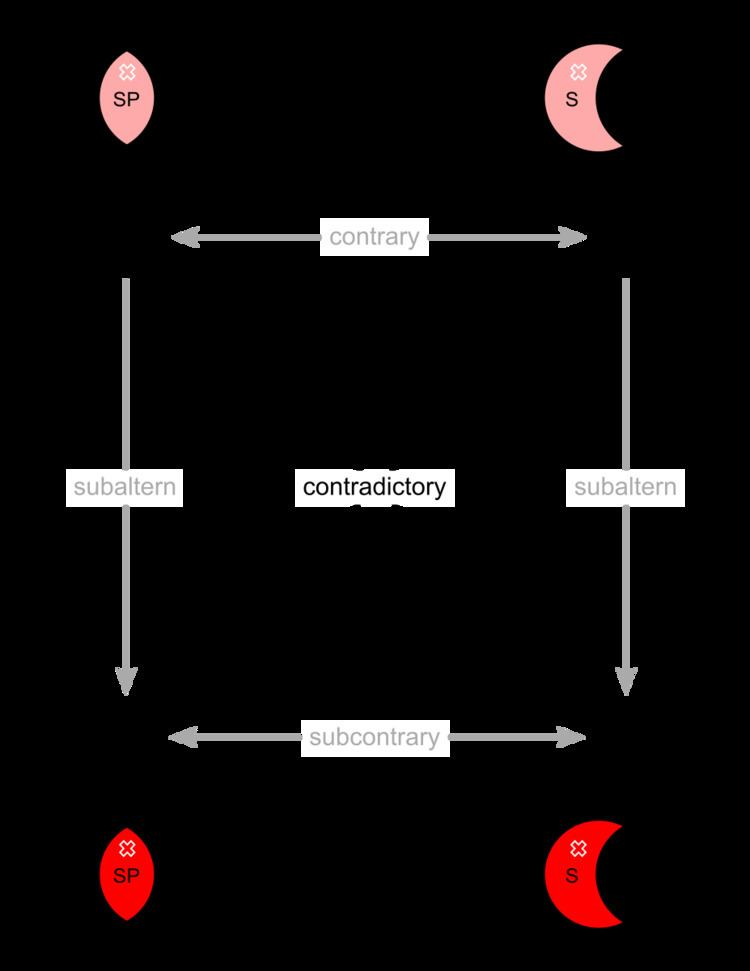# Empty domain

Updated onIn first-order logic the empty domain is the empty set having no members. In traditional and classical logic domains are restrictedly non-empty in order that certain theorems be valid. Interpretations with an empty domain are shown to be a trivial case by a convention originating at least in 1927 with Bernays and Schönfinkel (though possibly earlier) but oft-attributed to Quine 1951. The convention is to assign any formula beginning with a universal quantifier the value truth while any formula beginning with an existential quantifier is assigned the value falsehood. This follows from the idea that existentially quantified statements have existential import (i.e. they imply the existence of something) while universally quantified statements do not. This interpretation reportedly stems from George Boole in the late 19th century but this is debatable. In modern model theory, it follows immediately for the truth conditions for quantified sentences:

• A x ϕ ( x )  iff there is an  a A  such that  A ϕ [ a ]
• A x ϕ ( x )  iff every  a A  is such that  A ϕ [ a ]
• In other words, an existential quantification of the open formula φ is true in a model iff there is some element in the domain (of the model) that satisfies the formula; i.e. iff that element has the property denoted by the open formula. A universal quantification of an open formula φ is true in a model iff every element in the domain satisfies that formula. (Note that in the metalanguage, "everything that is such that X is such that Y" is interpreted as a universal generalization of the material conditional "if anything is such that X then it is such that Y". Also, the quantifiers are given their usual objectual readings, so that a positive existential statement has existential import, while a universal one does not.) An analogous case concerns the empty conjunction and the empty disjunction. The semantic clauses for, respectively, conjunctions and disjunctions are given by

• A ϕ 1 ϕ n ϕ i ( 1 i n ) , A ϕ i
• A ϕ 1 ϕ n ϕ i ( 1 i n ) , A ϕ i .
• It is easy to see that the empty conjunction is trivially true, and the empty disjunction trivially false.

Logics whose theorems are valid in every, including the empty, domain were first considered by Jaskowski 1934, Mostowski 1951, Hailperin 1953, Quine 1954, Leonard 1956, and Hintikka 1959. While Quine called such logics "inclusive" logic they are now referred to as free logic.

## References

Similar Topics
Hello Babies
Ernst Billgren
Kalifa Coulibaly
Topics